# Maharashtra Board Class 9 Maths Solutions Chapter 2 Real Numbers Practice Set 2.1

## Maharashtra State Board Class 9 Maths Solutions Chapter 2 Real Numbers Practice Set 2.1

Question 1.
Classify the decimal form of the given rational numbers into terminating and non-terminating recurring type.Solution:
i. Denominator = 5 = 1 x 5
Since, 5 is the only prime factor denominator.
the decimal form of the rational number $$\frac { 13 }{ 5 }$$ will be terminating type.

ii. Denominator = 11 = 1 x 11
Since, the denominator is other than prime factors 2 or 5.
∴ the decimal form of the rational number $$\frac { 2 }{ 11 }$$ will be non-terminating recurring type.

iii. Denominator = 16
= 2 x 2 x 2 x 2
Since, 2 is the only prime factor in the denominator.
∴ the decimal form of the rational number $$\frac { 29 }{ 16 }$$ will be terminating type.

iv. Denominator = 125
= 5 x 5 x 5
Since, 5 is the only prime factor in the denominator.
the decimal form of the rational number $$\frac { 17 }{ 125 }$$ will be terminating type.

v. Denominator = 6
= 2 x 3
Since, the denominator is other than prime factors 2 or 5.
∴ the decimal form of the rational number $$\frac { 11 }{ 6 }$$ will be non-terminating recurring type.

Question 2.
Write the following rational numbers in decimal form.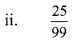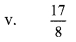Solution:
i. $$\frac { -5 }{ 7 }$$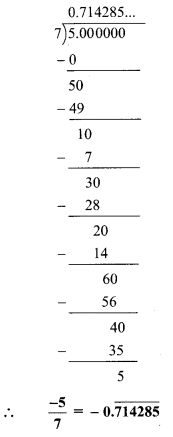ii. $$\frac { 9 }{ 11 }$$iii. √5iv. $$\frac { 121 }{ 13 }$$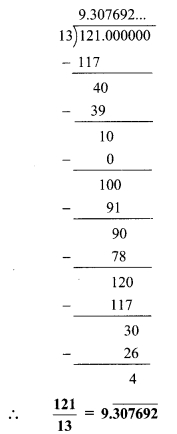v. $$\frac { 29 }{ 8 }$$Question 3.
Write the following rational numbers in $$\frac { p }{ q }$$ form.Solution:
i. Let x = $$0.\dot { 6 }$$ …(i)
∴ x = 0.666…
Since, one number i.e. 6 is repeating after the decimal point.
Thus, multiplying both sides by 10,
10x = 6.666…
∴ 10 x 6.6 …(ii)
Subtracting (i) from (ii),
10x – x = 6.6 – 0.6
∴ 9x = 6ii. Let x = $$0.\overline { 37 }$$
∴ x = 0.3737…
Since, two numbers i.e. 3 and 7 are repeating after the decimal point.
Thus, multiplying both sides by 100,
100x = 37.3737……
∴ 100x = $$37.\overline { 37 }$$ ……(ii)
Subtracting (i) from (ii),
100x – x = $$37.\overline { 37 }$$ – $$0.\overline { 37 }$$
∴ 99x = 37iii. Letx = $$3.\overline { 17 }$$ …(i)
∴ x = 3.1717…
Since, two numbers i.e. 1 and 7 are repeating after the decimal point.
Thus, multiplying both sides by 100,
100x = 317.1717…
∴ 100x= 317.17 …(ii)
Subtracting (i) from (ii),
100x – x = $$317.\overline { 17 }$$ – $$3.\overline { 17 }$$
∴ 99x = 314iv. Let x = $$15.\overline { 89 }$$ …….. (i)
∴ x = 15.8989…
Since, two numbers i.e. 8 and 9 are repeating after the decimal point.
Thus, multiplying both sides by 100,
100x= 1589.8989…
∴ 100x = $$1589.\overline { 89 }$$ …(ii)
Subtracting (i) from (ii),
100x – x = $$1589.\overline { 89 }$$ – $$15.\overline { 89 }$$
∴ 99x = 1574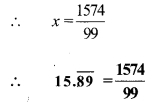v. Let x = $$2.\overline { 514 }$$
∴ x = 2.514514…
Since, three numbers i.e. 5, 1 and 4 are repeating after the decimal point.
Thus, multiplying both sides by 1000,
1000x = 2514.514514…
1000x = $$2514.\overline { 514 }$$ ….(ii)
Subtracting (i) from (ii),
1000x – x = $$2514.\overline { 514 }$$ – $$2.\overline { 514 }$$
∴ 999x = 2512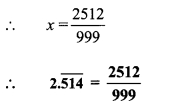Question 1.
How to convert 2.43 in $$\frac { p }{ q }$$ form ? (Textbook pg. no. 20)
Solution:
Let x = 2.43
In 2.43, the number 4 on the right side of the decimal point is not recurring.
So, in order to get only recurring digits on the right side of the decimal point, we will multiply 2.43 by 10.
∴ 10x = 24.3 …(i)
∴ 10x = 24.333…
Here, digit 3 is the only recurring digit. Thus, by multiplying both sides by 10, 100x = 243.333…
∴ 100x= 243.3 …(ii)
Subtracting (i) from (ii),
100x – 10x = 243.3 – 24.3
∴ 90x = 219